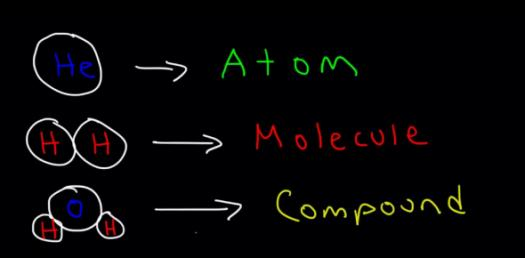# Chemistry Test On Atoms And Molecules! Trivia Quiz

18 Questions | Attempts: 293
ShareSettingsTest over chapter 6

• 1.
A compound is held together by
• A.

Chemical bonds

• B.

Physical bonds

• C.

Gravity

• D.

Magnetism

• 2.
Each molecule of hydrochloric acid, HCl, contains one atom of:
• A.

One atom of oxygen

• B.

Two atoms of chlorine

• C.

Two atoms of oxygen

• D.

One atom of chlorine

• 3.
In which substance do the molecules have the strongest attractions to one another?
• A.

Hydrogen, a gas

• B.

Sugar, a solid

• C.

Water, a liquid

• D.

Sulfuric acid, a liquid

• 4.
Atoms often join so that each atom will have
• A.

An outermost energy level that is full of electrons

• B.

An even number of electrons

• C.

More electrons that either protons or neutrons

• D.

An equal number of protons and electrons

• 5.
In a metallic bond, the nucleus of one atom is attracted by a nearby atom's
• A.

Nucleus

• B.

Energy structure

• C.

Electrons

• D.

Negative ion

• 6.
Solid ionic compounds have very high melting points because they
• A.

Are positively charged

• B.

Contain metallic elements

• C.

Are made of elements that are solid at room temperature

• D.

Contain chared ions that are locked tightly together

• 7.
The name for the compound with the formula CuBr2 would be written
• A.

Copper (I) bromide

• B.

Copper (II) bromide

• C.

Copper bromine

• D.

Copper (III) bromide

• 8.
Formaldehyde, CH20, and acetic acid, C2H4O2 have the same empirical formula but different
• A.

Kinds of anions

• B.

Kinds of cations

• C.

Molecular formulas

• D.

Kinds of atoms

• 9.
The simplest organic compound is
• A.

Methane

• B.

Salt

• C.

Aspirin

• D.

Table sugar

• 10.
Polymers are large organic molecules that are made of
• A.

Carbon and oxygen only

• B.

Cations

• C.

Anions

• D.

Repeating units

• 11.
A protein is a polymer that is made of
• A.

Amino acids

• B.

DNA

• C.

Nitrogen and carbon dioxide

• D.

Simple sugars

• 12.
• 13.
Formula units of salt, NaCI, contain equal numbers of___and____       .
• 14.
The distance between the nuclei of two bonded atoms is the             .
• 15.
A(n)____bond is formed by the attraction between positively charged metal ions and the_____electrons around them.
• 16.
A compound consisting of Br- and Cd2+ ions would be named
• 17.
The simplest formula for a covalent compound is its
• 18.
_______ are compounds that have repeating subunits.

## Related TopicsBack to top
×

Wait!
Here's an interesting quiz for you.